# Train Deep Learning-Based Sampler for Motion Planning

This example demonstrates how to train a deep learning-based sampler to speed up path planning using sampling-based planners like RRT (rapidly-exploring random tree) and RRT*.

The classical sampling-based planners such as RRT and RRT* rely on generating samples from a uniform distribution over a specified state space. However, these planners typically restrict the actual robot path to a small portion of the state space. The uniform sampling causes the planner to explore many states which do not have an impact on the final path. This causes the planning process to become slow and inefficient, especially for state spaces with a large number of dimensions.

You can train a deep learning network to generate learned samples that can bias the path towards the optimal solution. This example implements the approach proposed by Ichter et al. in their paper titled "Learning Sampling Distributions for Robot Motion Planning". This approach implements a Conditional Variation Autoencoder (CVAE) that generates learned samples for a given map, start state, and goal state.

The learned sampling alone cannot guarantee the probabilistic completeness and asymptotic optimality that uniform sampling does. Hence, you can mix both learned samples and uniform samples in a certain proportion `λ`, to bias the planner towards the optimal solution while also guaranteeing to find a solution. `λ=0` indicates pure uniform sampling, `λ=1` indicates pure learned sampling, and `0<λ<1` indicates the combination of both.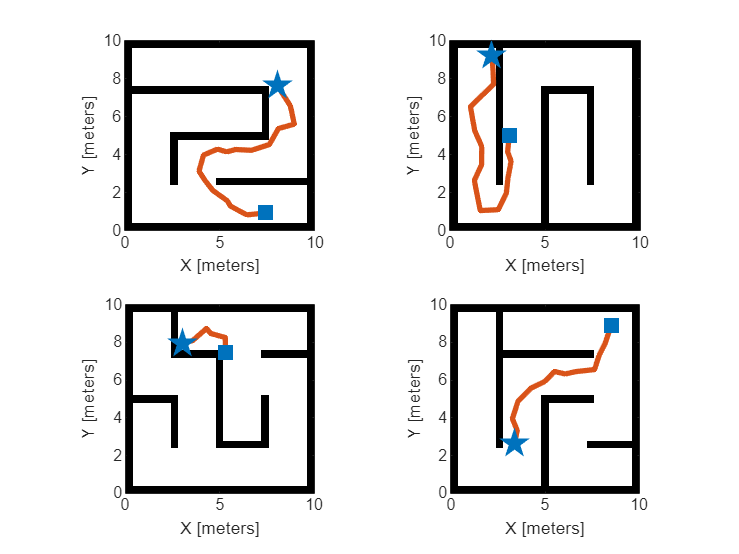Load the pretrained network from the mat file `CVAESamplerTrainedModel.mat`. The network was trained using the dataset `MazeMapDataset.mat`. If you want to train the network, set the `doTraining` to `true`.

```doTraining=false; if ~doTraining load("CVAESamplerTrainedModel","encoderNet","decoderNet") end```

Load the dataset from the mat file `MazeMapDataset.mat`. The dataset contains 2000 maze maps and their corresponding start states, goal states, and path states.

`load("MazeMapDataset","dataset","mapParams")`

#### Dataset Generation

The dataset was generated using the `examplerHelperGenerateData` function. Note that the dataset generation took more than 90 minutes to complete for the settings used in the helper function. The time taken for dataset generation may vary for your system. To train for different types of maps, you can replace or modify the `examplerHelperGenerateData` function.

The following code snippet from the `examplerHelperGenerateData` function shows the generation of maps using the `mapMaze` function. You can modify the settings for the `mapMaze` function or replace them with different map generation function.

```%% Generate maps % Set random seed rng("default"); % Number of maps numMaps = 2000; % Maze map parameters mapSize = 10; % Map size in meters (assume height = weight) gridSize = 25; % Number of grid cells (assume height = weight) passageWidth = 5; % in cells wallThickness = 1; % in cells mapRes = gridSize/mapSize; % map resolution (cells per meter) % Generate maps for k=1:numMaps maps{k} = mapMaze(passageWidth,wallThickness, ... MapSize=[mapSize,mapSize], ... MapResolution=mapRes); end ```

The following code snippet from the `examplerHelperGenerateData` function shows the set of start and goal states chosen for the problem.

```% Randomly sample two different start and goal states from this startGoalStates = [1, 1, 0; 9, 9, 0; 9, 1, 0; 1, 9, 0]; ```

The following code snippet from the `examplerHelperGenerateData` function shows the optimal paths generation using the `plannerRRTStar` object. You can modify the settings to get different optimal paths.

```planner = plannerRRTStar(stateSpace, stateValidator); planner.ContinueAfterGoalReached = true; % optimize planner.MaxConnectionDistance = 1; planner.GoalReachedFcn = @examplerHelperCheckIfGoalReached; planner.MaxIterations = 2000; ```

#### Visualize Dataset

```figure for i=1:4 subplot(2,2,i) % Select a random map ind = randi(length(dataset)); exampleHelperPlotData(dataset(ind).maps,dataset(ind).startStates,dataset(ind).goalStates, ... navPath(stateSpaceSE2,dataset(ind).pathStates)); end```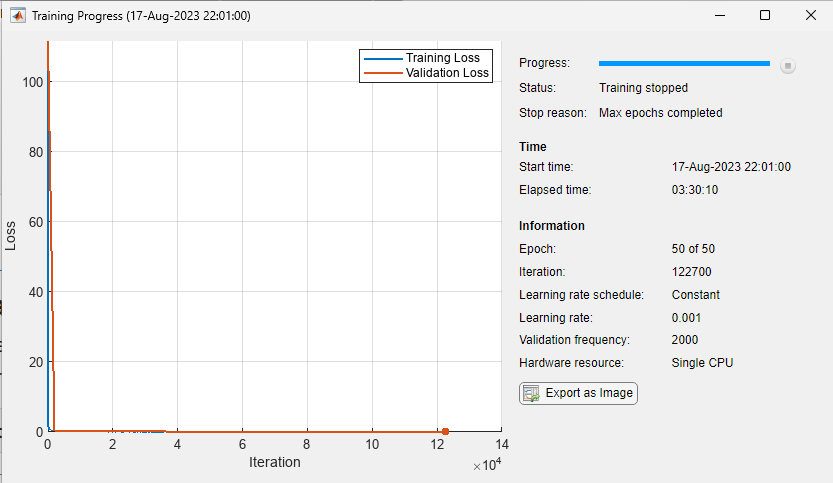### Prepare Data for Training

#### Compress Maps

In the real-world scenario, the occupancy maps can be quite large, and the map is usually sparse. You can compress the map to a compact representation using the `trainAutoencoder` (Deep Learning Toolbox) function. This helps training loss to converge faster for the main network during training in the Train Deep Learning Network section.

Load the pretrained autoencoder model from the mat file `MapsAutoencoder.mat`.

`load("MazeMapAutoencoder","mapsAE")`

The `exampleHelperCompressMaps` function was used to train the autoencoder model for the random maze maps. In this example, the map of size `25x25=625` is compressed to `50`. Hence, `workSpaceSize` is set to `50` in the Define CVAE Network Settings section. To train for a different setting, you can replace or modify the `exampleHelperCompressMaps` function.

#### Process Dataset

You need to process the loaded dataset into the format required for training the network using the `exampleHelperProcessData` function.

The most crucial step in data processing is to make sure that the scaling used for the dataset is in the range of `[0,1]` or `[-1,1]`.

• The map data is in the form of a binary occupancy matrix, and it is already in the range of `[0,1]`.

• Normalize the position `X`, `Y` of the states to `[0,1]` by dividing them with the `mapSize` parameter.

• Normalize the orientation `theta` to `[-1,1]` by dividing them with `pi`.

Use the `exampleHelperNormalizeStates` function to normalize the states data. During the prediction, denormalize the states data using the `exampleHelperDenormalizeStates` function.

The next data processing step is to divide the state samples into multiple dependent sets. Choose these sample sets such that they are well dispersed. At each training step, the network will train on multiple samples drawn from these sets. The network will learn to represent the samples along the solution trajectory through multiple distributions.

Specify the number of dependent sets using `numDependentSets`. Specify the `split` that corresponds to the fraction of the dataset used for the training. Then use the remaining fraction `(1-split)` for evaluation.

```split = 0.9; numDependentSets = 5; [trainCondition,trainStates,testCondition,testStates] = exampleHelperProcessData(dataset,mapsAE,numDependentSets,split);```

### Define Network Architecture

The deep learning network used to generate learned samples in this example is based on CVAE. The CVAE is an extension of a Variational Autoencoder (VAE) which is a generative model used to "generate data" based on random Gaussian input. See Train Variational Autoencoder (VAE) to Generate Images (Deep Learning Toolbox) example to know how VAE works. The CVAE takes an additional input called "condition" so that the data is generated from a conditional probability distribution.

In this example, "data generated" corresponds to the learned state samples. The "condition" corresponds to the workspace information of the robot (occupancy map), start states, and goal states. The network learns the probability distribution of the path "states" conditioned on the "condition" inputs.

The CVAE works differently during the training and prediction (or deployment) phases:

• In the training phase, the encoder takes input state $x$, input condition $y$, and computes the latent state $z$. The KL (Kullback–Leibler) divergence loss at the output of the encoder will try to match the distribution of $z$ with the normal distribution $N\left(0,I\right)$. The decoder takes the input condition $y$, the latent state $z$, and computes the predicted states $x$. The mean squared loss at the output of the decoder will try to make the predicted state $\underset{}{\overset{ˆ}{x}}$ the same as the input state $x$.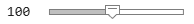• During the prediction phase use only the decoder. The normal distribution $N\left(0,I\right)$ provides the input condition $y$ for a specified map, start, goal, and input latent $z$. The decoder predicts the learned samples which the sampling-based planner can use. You can query a large number of states in one step, and this will be faster on a GPU.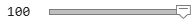#### Define CVAE Network Settings

Specify these settings for creating the CVAE network:

• The `stateSize` is the size of the SE(2) state vector `[X,Y,theta]`.

• The `workspaceSize` can be cell values of the maze map or the compressed representation. In this example, you can choose the compressed representation of the map for better training convergence.

• The `latentStateSize` is the number of dimensions of multivariate Gaussian distribution.

• The `conditionSize` is sum of `workspaceSize`, start `stateSize` and goal `stateSize`.

```stateSize = 3; workspaceSize = 50; latentStateSize = 4; conditionSize = workspaceSize + 2*stateSize; ```

#### Create CVAE Encoder Network

The CVAE encoder network is a neural network that consists of fully connected layers with the ReLU (Rectified Linear Unit) activation function layer and dropout layers in between. The dropout layers help to reduce overfitting and achieve better generalization. The input layer of the encoder takes the concatenated condition $y$ and state $x$ vectors. The final layer of the encoder computes the mean and standard deviation of the latent state vector $z$, using the `exampleHelperSamplingLayer` function.

```% Hidden sizes of fully connected layers in the encoder network encoderHiddenSizes = [512, 512]; % Probability values for the dropout layers prob = [0.10, 0.01]; % Create layers encoderLayers = featureInputLayer(numDependentSets*stateSize+conditionSize, Name="encoderInput"); for k=1:length(encoderHiddenSizes) encoderLayers(end+1) = fullyConnectedLayer(encoderHiddenSizes(k)); %#ok<*SAGROW> encoderLayers(end+1) = reluLayer; encoderLayers(end+1) = dropoutLayer(prob(k)); end encoderLayers(end+1) = fullyConnectedLayer(2*latentStateSize); encoderLayers(end+1) = exampleHelperSamplingLayer(Name="encoderOutput"); % Create layer graph and dlnetwork object encoderGraph = layerGraph(encoderLayers); % Create this network only when doTraining=true if doTraining encoderNet = dlnetwork(encoderGraph); end```

#### Create CVAE Decoder Network

The CVAE decoder network is a neural network that consists of fully connected layers with ReLU and dropout layers in between. The input layer of the decoder takes the concatenated condition $y$ and the latent state $z$ vectors. The final layer of the decoder computes the predicted states $\underset{}{\overset{ˆ}{x}}$.

```% Hidden sizes of fully connected layers in the decoder network decoderHiddenSizes = [512 512]; % Probability values for the dropout layers prob = [0.10 0.01]; % Create layers decoderLayers = featureInputLayer(conditionSize+latentStateSize,Name="decoderInput"); for k=1:length(decoderHiddenSizes) decoderLayers(end+1) = fullyConnectedLayer(decoderHiddenSizes(k)); %#ok<*SAGROW> decoderLayers(end+1) = reluLayer; decoderLayers(end+1) = dropoutLayer(prob(k)); end decoderLayers(end+1) = fullyConnectedLayer(numDependentSets*stateSize,Name="decoderOutput"); % Create layer graph decoderGraph = layerGraph(decoderLayers); % Create this network only when doTraining=true if doTraining decoderNet = dlnetwork(decoderGraph); end```

### Train Deep Learning Network

#### Training Options

Specify these training options for training the deep learning network:

• Set the number of epochs to `100`.

• Set the mini-batch size for training to `32`.

• Set the learning rate to `1e-3`.

• Set the beta weight for KL divergence loss to `1e-4`. See Model Loss Function.

• Set the weight for the mean squared error loss to `[1,1,0.1]`. See Model Loss Function.

```options = struct; options.NumEpochs = 100; options.TrainBatchSize = 32; options.LearningRate = 1e-3; options.Beta = 1e-4; options.Weight = [1,1,0.1]; ```

#### Train Network

Use the exampleHelperTrainCVAESampler function for training the neural network which is based on the concept of custom training loops, see Define Custom Training Loops, Loss Functions, and Networks (Deep Learning Toolbox). The neural network was trained using a NVIDIA GeForce GPU with 8 GB graphics memory. Training this network for 100 epochs took approximately 11 hours. The training time may vary for your system.

In this example, the provided pretrained model `CVAESamplerTrainedModel.mat` loads by default. To train the model with a custom network and custom dataset, set `doTraining` to `true` in the Load Pretrained Network section.

```if doTraining % For reproducibility rng("default") % Create mini-batch queue for training trainData = combine(arrayDatastore(trainCondition),arrayDatastore(trainStates)); mbqTrain = minibatchqueue(trainData,MiniBatchSize=options.TrainBatchSize, ... OutputAsDlarray=[1,1],MiniBatchFormat={'BC','BC'}); % Train the CVAE sampler model figure(Name="Training Loss"); [encoderNet,decoderNet] = exampleHelperTrainCVAESampler(encoderNet,decoderNet, ... @lossCVAESampler,mbqTrain, ... options); end```

### Predict Using New Data

Use the trained network to generate learned samples for the part of the dataset kept aside for prediction. In the Process Dataset section, set `split` to `0.9`, so you have 10% of the dataset for prediction.

#### Prepare Test Set

```% For reproducibility rng("default") % Prepare test mini-batches testData = combine(arrayDatastore(testCondition),arrayDatastore(testStates)); mbqTest = minibatchqueue(testData, MiniBatchSize=1,... OutputAsDlarray=[1,1],MiniBatchFormat={'BC','BSC'}); shuffle(mbqTest) ```

#### Generate Learned Samples

Use the exampleHelperGenerateLearnedSamples function to generate the learned samples. Press the `Run` button below to generate learned samples for different maps at each time. You can adjust the `lambda` value to visualize the combination of learned samples and uniform samples.

```% Press Run button to visualize results for new maps% Vary lambda to visualize results for different ratios of learned samples to total samples lambda =1; % Number of samples to be generated numSamples = 2000; if ~hasdata(mbqTest) reset(mbqTest) end % Generate samples for different test maps figure(Name="Prediction"); for k = 1:4 [mapMatrix,start,goal,statesLearned] = exampleHelperGenerateLearnedSamples(encoderNet, ... decoderNet,mapsAE,mbqTest,numDependentSets, ... mapParams.mapSize,numSamples,lambda); % Visualize the samples map = binaryOccupancyMap(mapMatrix,mapParams.mapRes); subplot(2,2,k) exampleHelperPlotData(map,start,goal,statesLearned); end```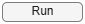### Conclusion

This example shows how to train a deep learning network to generate learned samples for sampling-based planners such as RRT and RRT*. It also shows the data generation process, deep learning network setup, training, and prediction. You can modify this example to use with custom maps and custom datasets. Further, you can extend this for applications like manipulator path planning, 3-D UAV path planning, and more.

To augment sampling-based planners with the deep learning-based sampler to find optimal paths efficiently, See Accelerate Motion Planning with Deep-Learning-Based Sampler example.

### Supporting Functions

#### Model Loss Function

Use the `lossCVAESampler` function for training the deep learning network in the Train Deep Learning Network section. The loss function consists of two components: The Define Network Architecture section describes the KL divergence loss and mean squared. Train Variational Autoencoder (VAE) to Generate Images (Deep Learning Toolbox) example also describes these losses.

```function [loss,gradientsEncoder,gradientsDecoder] = lossCVAESampler(encoderNet,decoderNet,condition,state,beta,weight) % lossCVAESampler Define losses for the CVAE network % Predict latent states from encoder [z,zMean,zLogVarSq] = forward(encoderNet,vertcat(state,condition)); % Predict state from decoder statePred = forward(decoderNet,vertcat(condition,z)); %% KL diveregence loss klloss = exp(zLogVarSq) + zMean.^2 - zLogVarSq -1; % Reduce sum over zdim klloss = sum(klloss,1); % Reduce mean over batch klloss = mean(klloss); % Weighting term for KL loss klloss = klloss*beta; %% Reconstruction loss reconLoss = (state-statePred).^2; % Apply weight vector to state vector numSets = size(reconLoss,1)/length(weight); weight = repmat(weight,numSets,1); reconLoss = reconLoss.* weight; % Reduce mean over batches reconloss = mean(reconLoss,1); % Reduce mean over state vector dimensions reconloss = mean(reconloss); % Total loss loss = klloss + reconloss; % Gradients [gradientsEncoder,gradientsDecoder] = dlgradient(loss,encoderNet.Learnables,decoderNet.Learnables); % Convert loss to double loss = double(loss); end```

### Bibliography

1. Ichter, Brian, James Harrison, and Marco Pavone. “Learning Sampling Distributions for Robot Motion Planning.” In 2018 IEEE International Conference on Robotics and Automation (ICRA), 7087–94. Brisbane, QLD: IEEE, 2018. https://doi.org/10.1109/ICRA.2018.8460730.